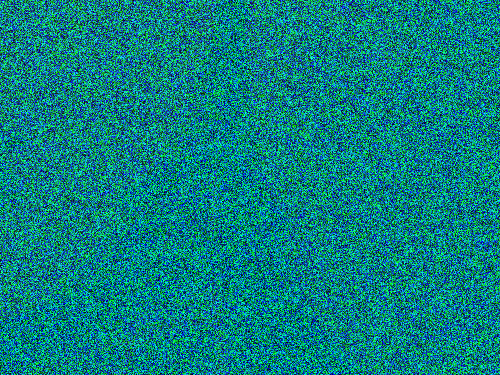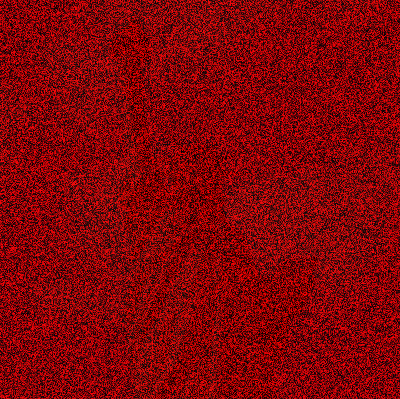Nifty Image Puzzles

Nick Parlante, Nifty Assignments 2011

The "image puzzle" is a short, nifty Media Computation exercise. You have 2-d pixel data for image of a mystery thing, however the pixels are messed up in some way. The students write code to fix the image, and so reveal the mystery object. What makes this assignment nifty is that the code required is quite simple -- basically calling a couple methods, passing in the right values -- yet the puzzle problem is engaging and the output is neat. It's also a pretty short exercise however you slice it. I got his idea originally from David Malan.

I use this in CS101, a CS0 / CS Principles type course. In this course I provide the "loop to touch every pixel", so the students just write the change-one-pixel code, essentially calling a few setXXX() methods with the right values to fix the image.

Three Puzzles - How to Adopt

It's a nice simple assignment idea; as soon as you see it, you'll understand how it works. Part of the value of this page is that three versions, complete with PNG files and problem statements are done, so it's very ready to adopt. Using the CS101 code-in-browser infrastructure below, the puzzles will just run embedded in this page. You can just point students here, or copy the puzzle PNG files to solve it your own way. The original images were public domain, and I hereby place these PNG files in the public domain.

1. Iron Image PuzzleThe iron-puzzle.png image is a puzzle; it contains an image of something famous, however the image has been distorted. The famous object is in the red values, however the red values have all been divided by 10, so they are too small by a factor of 10. The blue and green values are all just meaningless random values ("noise") added to obscure the real image. You must undo these distortions to reveal the real image. First, set all the blue and green values to 0 to get them out of the way. Look at the result .. if you look very carefully, you may see the real image, although it is very very dark (way down towards 0). Then multiply each red value by 10, scaling it back up to approximately its proper value. What is the famous object?

 im = new SimpleImage("iron-puzzle.png"); for (x = 0; x < im.getWidth(); x++) { for (y = 0; y < im.getHeight(); y++) { // code for each x,y pixel here } } print(im);

2. Copper Image PuzzleThe copper-puzzle.png image is a puzzle -- it shows something famous, however the image has been distored. The true image is in the blue and green values, however all the blue and green values have all be divided by 20, so the values are very small. The red values are all just random numbers, noise added on top to obscure things. Undo these distortions to reveal the true image.

First, set the red values to 0 to get that of the way. You may be able to see the image very faintly at this point, but it is very dark. Then multiply the blue and green values by 20 to get them back approximately to their proper values. What is the famous object?

 im = new SimpleImage("copper-puzzle.png"); for (x = 0; x < im.getWidth(); x++) { for (y = 0; y < im.getHeight(); y++) { // your code here } } print(im);

3. West Image Puzzle

The west-puzzle.png image is a puzzle. It shows something famous, however the image has been distorted. Use if-logic along with other pixel techniques to recover the true image. The true image is exclusively in the blue values, so set all red and green values to 0. The hidden image is encoded using only the blue values that are less than 16 (that is, 0 through 15). If a blue value is less than 16, multiply it by 16 to scale it up to its proper value. Alternately if a blue value in the encoded image is 16 or more, it is random garbage and should be ignored (interpreted as 0). This should yield the recovered image, but all in the blue channel. As a final fix, the image should be in the red channel to look more correct, so change your code to also move the values from the blue channel to the red channel.

 im = new SimpleImage("west-puzzle.png"); for (x = 0; x < im.getWidth(); x++) { for (y = 0; y < im.getHeight(); y++) { // your code here } } print(im);